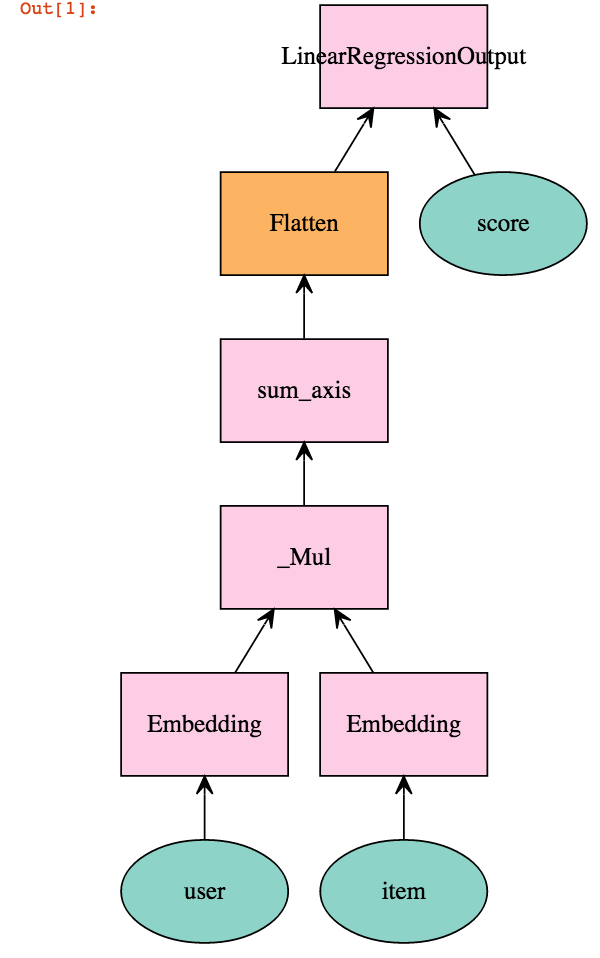# How to visualize Neural Networks as computation graph

Here, we'll demonstrate how to use `mx.viz.plot_network` for visualizing your neural networks. `mx.viz.plot_network` represents the neural network as a computation graph consisting of nodes and edges. The visualizations make clear which nodes correspond to inputs, where the computation starts, and which correspond to output nodes, from which the result can be read.

## Prerequisites

You need the Jupyter Notebook and Graphviz libraries to visualize the network. Please make sure you have followed installation instructions in setting up above dependencies along with setting up MXNet.

## Visualize the sample Neural Network

`mx.viz.plot_network` takes Symbol, with your Network definition, and optional node_attrs, parameters for the shape of the node in the graph, as input and generates a computation graph.

We will now try to visualize a sample Neural Network for linear matrix factorization: - Start Jupyter notebook server ```bash \$ jupyter notebook ``` - Access Jupyter notebook in your browser - http://localhost:8888/. - Create a new notebook - "File -> New Notebook -> Python 2" - Copy and run below code to visualize a simple network.

``````import mxnet as mx
user = mx.symbol.Variable('user')
item = mx.symbol.Variable('item')
score = mx.symbol.Variable('score')

# Set dummy dimensions
k = 64
max_user = 100
max_item = 50

# user feature lookup
user = mx.symbol.Embedding(data = user, input_dim = max_user, output_dim = k)

# item feature lookup
item = mx.symbol.Embedding(data = item, input_dim = max_item, output_dim = k)

# predict by the inner product, which is elementwise product and then sum
net = user * item
net = mx.symbol.sum_axis(data = net, axis = 1)
net = mx.symbol.Flatten(data = net)

# loss layer
net = mx.symbol.LinearRegressionOutput(data = net, label = score)

You should see computation graph something like the following image: